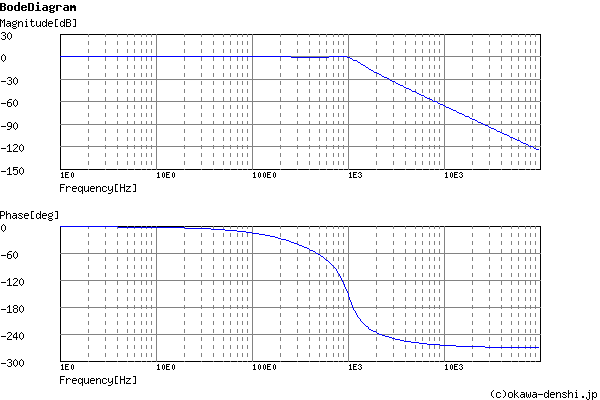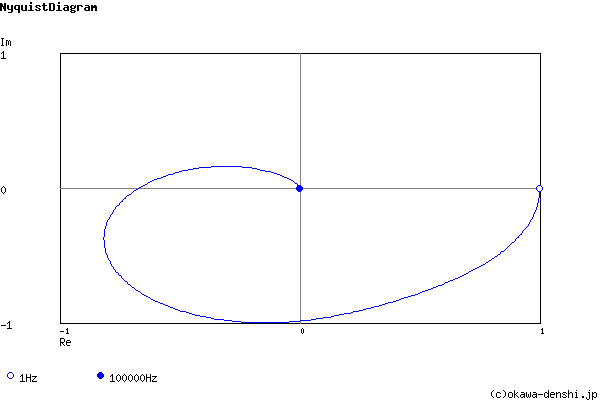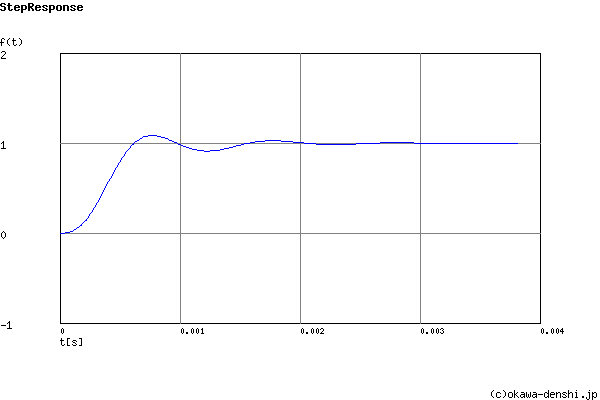# (Sample) 3rd order Sallen-Key Low-pass Filter Design Tool - Result -

Calculated the Transfer Function for the 3rd order Sallen-Key Low-pass filter, displayed on graphs, showing Bode diagram, Nyquist diagram, Impulse response and Step response.

## 3rd order Sallen-Key filter

 Vi→→Vo
(Sample)Transfer Function:
 G(s)= 133362301759 s3+6607.88013729s2+50305327.1219s+133362301759

R1 = 9.1kΩ
R2 = 68kΩ
R3 = 36kΩ
C1 = 0.033uF
C2 = 0.015uF
C3 = 680pF

#### Equivalent block diagram:

 Vi(s)→ 2πfc1 s+2πfc1 → (2πfc2)2 s2+2ζ(2πfc2)s+(2πfc2)2 →Vo(s)
Cut-off frequency fc1, fc2 of equivalent block diagram:
fc1 = 538.768389471[Hz]
fc2 = 998.954448652[Hz]
Damping ratio ζ of equivalent block diagram:
ζ = 0.256722615545

#### Pole(s)

p = -256.454198869 +965.474616116i[Hz]
|p|= 998.954448652[Hz]
p = -538.768389471[Hz]
|p|= 538.768389471[Hz]
p = -256.454198869-965.474616116i[Hz]
|p|= 998.954448652[Hz]#### Phase margin

pm= 42.7[deg] (f =941.5[Hz])

#### Oscillation frequency

f = 965.474616116[Hz]

#### Overshoot (in absolute value)

The 1st peak  gpk = 1.09 (t =0.00076[sec])
The 2nd peak  gpk = 0.91 (t =0.0012[sec])
The 3rd peak  gpk = 1.03 (t =0.0018[sec])

g(∞) = 1

### Select filter type

Set parameters of the equivalent block diagram
 1st filter: fc1=Hz 2nd filter: fc2=Hz Damping ratio ζ=

Butterworth filter
Cut-off frequency fc=Hz

Chebyshev filter
Characteristic frequency fc=Hz
Gain ripple gr=dB

 C1 = F C2 = F
C1, C2 is optional. But when setting these capacitances, C1 and C2 of both are needed.

Select Capacitor Sequence:
Select Resistor Sequence:

### Frequency analysis

Bode diagram
Phase  Group delay
Nyquist diagram
Pole, zero
Phase margin
Oscillation analysis
Analysis on frequency range:
f1=∼f2=[Hz] (optional)

### Transient analysis

Step response
Impulse response
Overshoot
Final value of the step response
Analysis on time range:
0∼[sec] (optional)

# Frequency analysis# Transient analysis